Courses

# Activated Complex Theory Of Bimolecular Reaction Or Transition State Theory Or Eyring Equation Chemistry Notes | EduRev

## Chemistry : Activated Complex Theory Of Bimolecular Reaction Or Transition State Theory Or Eyring Equation Chemistry Notes | EduRev

The document Activated Complex Theory Of Bimolecular Reaction Or Transition State Theory Or Eyring Equation Chemistry Notes | EduRev is a part of the Chemistry Course Physical Chemistry.
All you need of Chemistry at this link: Chemistry

Activated complex Theory of Bimolecular Reaction or Transition state Theory or Eyring Equation

The activated complex forms between reactants as they collide. The difference between the energy of the activated complex and the energy of the reactants is the activation energy, Ea.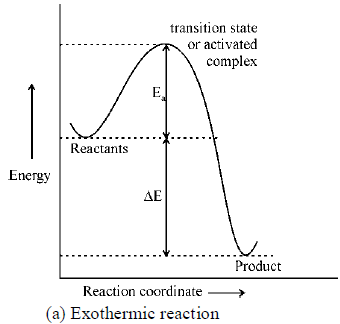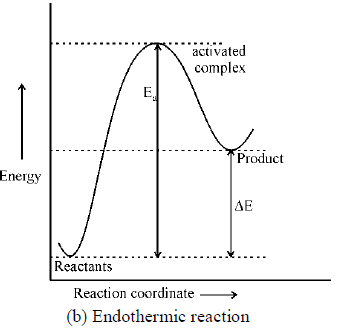(a) Exothermic react ion (b) Endothermic reaction According to Eyring the equilibrium is between reactants and the activated complex.
Consider A and B react to form an activated complex that undergoes decay, resulting in product formation.
The activated complex represents the system at the transition state.
This complex is stable.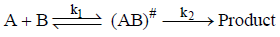where (AB)# is the act ivated complex and k1 is the equilibrium constant between reactants and activated complex.
If (AB)# one of the vibrat ional degrees of freedo m has become a translat ional degree of freedo m.
From the classical mechanics,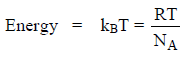kB = Boltzmann constant

from the quantum mechanics,
energy = hv

than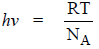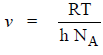The vibrat ional frequency v is the rate at which the activated complex mo ve across the energ y barrier i.e. the rate constant k2 is ident ified by v.
Then the reaction is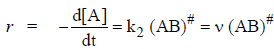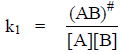⇒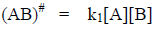then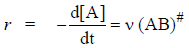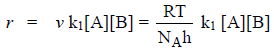for conventional rate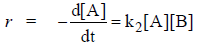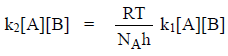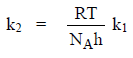i.e. k2 = v × k1
k2 = frequency × k1                                            …(1)

where k1 = equilibrium constant = keq

Relation between k1 and ΔG#:
k1 = equilibrium constant =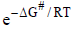&  ΔG# = ΔH# - TΔS#
where ΔG#, ΔH# & ΔS# are the standard free energy o f act ivat ion, enthalpy o f act ivat ion and entropy of activation.

k2 = keq × frequency = k1× frequency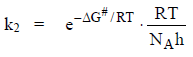…(2)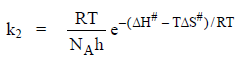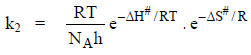…(3)
∵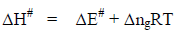∴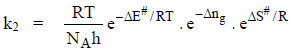…(4)

and  Δng =  difference is number of mo les between transit ion state and reactant

Relation between ΔE# and Ea

We know that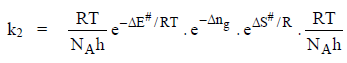On taking log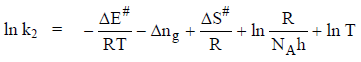On differentiate above equation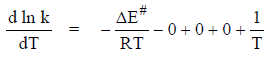or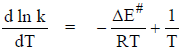and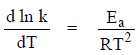(from arrhanius equation)
then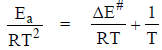Ea = ΔE# + RT                                                     …(5)

Relation between E0 & ΔE#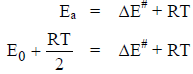E0 = Collision energy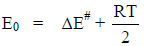Value of A, using above equations From Eyring theory and Arrhenius theory we have

rate constant =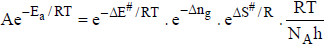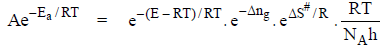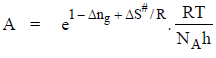…(6)

Problem.  Consider the decomposition of

NOCl, 2NOCl(g) → 2NO(g) + Cl2(g)

The Arrhenius parameters for this reaction are A = 1.00 × 1013 M–1 s–1 and Ea = 104 kJ mol–1. Calculate ΔH# and ΔS# for this reaction with T = 300 K.
Sol.
We know that

ΔH# = ΔE# + ΔngRT

where Δng = difference in number of moles between act ivated complex and reactant.

ΔH# = ΔE# + (-1) RT
ΔH# = ΔE# - RT

&                    Ea = ΔE# + RT

then               ΔE# = Ea - RT
∴                    ΔH# = ΔE# + RT = Ea - RT - RT = Ea - RT
ΔH# = 104 kJ mol-1 - 2(8.314 J mol-1 K-1) (300 K)
= 99.0 kJ mol-1
We know that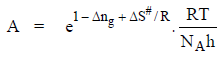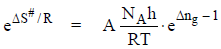[Δng = -1]
Taking log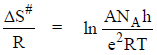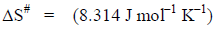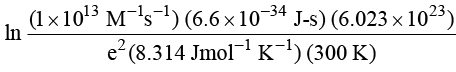ΔS# = -12.7 J mol-1 K-1

Note: for unimolecular      Δng = 0

for bimolecular Δng = -1
for trimolecular            Δng = -2
&                                 ΔH# = ΔE# + ΔngRT                                [∵ Ea = ΔE# + RT]4
= Ea - RT + ΔngRT
⇒                                 Ea = ΔH# + RT - ΔngRT
for unimolecular            Ea = ΔH# + RT
for bimolecular              Ea = ΔH# + 2RT
for trimolecular              Ea = ΔH# + 3RT

The Pre-equilibrium Approximation: Consider the following reaction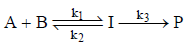(i) First, equilibrium between the reactants and the intermediate is maintained during the course of the reaction. (ii)  The intermediate undergoes decay to form product.
Then the rate law expression is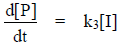∵ I is in equilibrium wit h the reactant then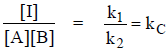= equilibrium constant

[I] = kC [A][B]

∴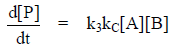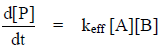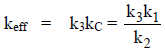The Lindemann Mechanism

Lindemann mechanism for unimolecular reactions involves two steps. First reactants acquire sufficient energy to undergo reaction through a bimolecular collision.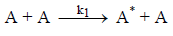In this,  A* is the act ivated reactant and undergoes one of two reactions.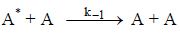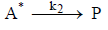Then, rate of product formation is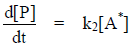& rate of formation of A*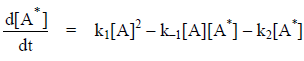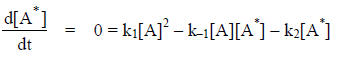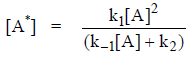Then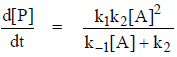It state that the observed order dependence on [A] depends on the relative magnitude of k–1[A] versus k2. At high reactant concentration, k–1[A] > k2 and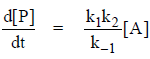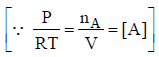i.e. the product formation is first order at high pressure. At low reactant concentration k2 > k–1[A] and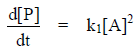i.e. at low pressure, the rate of formation product is second order in [A].
Then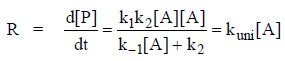kuni is the apparent rate constant for the reaction defined as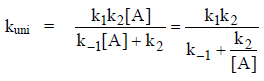and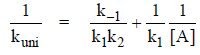when k–1[A] >> k2 i.e. at high concentration kuni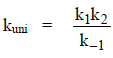when k–1[A] << k2 i.e. at low concentration kuni = k1[A]

kuni = k1[A]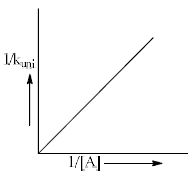Offer running on EduRev: Apply code STAYHOME200 to get INR 200 off on our premium plan EduRev Infinity!

## Physical Chemistry

66 videos|94 docs|32 tests

,

,

,

,

,

,

,

,

,

,

,

,

,

,

,

,

,

,

,

,

,

;